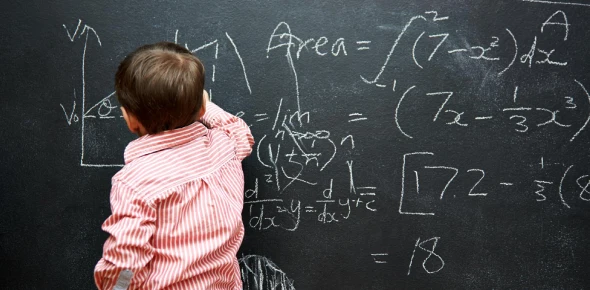# Grade 4 Math Quiz Bee

Approved & Edited by ProProfs Editorial Team
The editorial team at ProProfs Quizzes consists of a select group of subject experts, trivia writers, and quiz masters who have authored over 10,000 quizzes taken by more than 100 million users. This team includes our in-house seasoned quiz moderators and subject matter experts. Our editorial experts, spread across the world, are rigorously trained using our comprehensive guidelines to ensure that you receive the highest quality quizzes.
| By Boyetme
B
Boyetme
Community Contributor
Quizzes Created: 3 | Total Attempts: 32,217
Questions: 10 | Attempts: 4,031SettingsThese were the questions my Grade 4 students answered during the Math Quiz Bee. Questions 1 to 4 were Easy questions (1 point each). Questions 5 to 7 were Average questions (3 points each). Questions 8 to 10 were Difficult questions (5 points each).

• 1.

• 2.

### What number completes the sentence?      28542 + _______ = 70789

Explanation
To find the missing number, we need to subtract 28,542 from 70,789. The difference is 42,247, which is the missing number that completes the sentence.

Rate this question:

• 3.

### Write the number below in words:      15 319

Explanation
The given number, 15,319, can be written as "fifteen thousand, three hundred and nineteen" in words.

Rate this question:

• 4.

• 5.

### Billy sold 4 034 cakes on Sunday. He sold 1 429 cakes on Monday. How many more cakes did he sell in Sunday than Monday?

Explanation
Billy sold 4,034 cakes on Sunday and 1,429 cakes on Monday. To find out how many more cakes he sold on Sunday than Monday, we need to subtract the number of cakes sold on Monday from the number of cakes sold on Sunday. Therefore, the difference is 4,034 - 1,429 = 2,605 cakes.

Rate this question:

• 6.

C.
• 7.

### Douglas gave away 8 107 toys during a party. There were 3 297 toys left.. How many toys were there at first?

11 404 toys
Explanation
Since Douglas gave away 8,107 toys during the party, and there were 3,297 toys left, we can calculate the total number of toys at first by adding the toys given away and the toys left. Therefore, the total number of toys at first was 8,107 + 3,297 = 11,404 toys.

Rate this question:

• 8.

### I slept late last night and woke up at 5:45 am today. If I had 6 hours of sleep last night, what time did I sleep?

11:45 pm
Explanation
Based on the information given, the person slept for 6 hours last night and woke up at 5:45 am today. To determine the time they slept, we need to subtract 6 hours from the time they woke up. By doing so, we get the time of 11:45 pm as the time they went to sleep.

Rate this question:

• 9.

### Complete the pattern: _______ , 57 326 , 54 428 , 51 530 , 48 632 , 45 734 , _______ Separate the two numbers with a comma.

60 224 , 42 836
Explanation
The pattern in the given sequence is that the first number is decreasing by 3,000 each time, while the second number is increasing by 2,102 each time. Therefore, to complete the pattern, the missing numbers would be 60 224 (57 326 - 3,000) and 42 836 (45 734 - 2,102).

Rate this question:

• 10.

### Using all the digits 2, 5, 6, 7 and 9, form two numbers that have the greatest difference between them. What is the difference?

71 973
Explanation
To form two numbers with the greatest difference, we need to maximize the value of one number and minimize the value of the other. By arranging the digits in descending order, we get 9, 7, 6, 5, 2. Using these digits, we can form the number 9,762 and the number 5,297. The difference between these two numbers is 9,762 - 5,297 = 4,465.

Rate this question:

Related TopicsBack to top